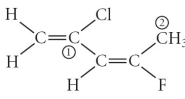# Problem: Consider the molecule below. Determine the hybridization at each of the 2 labeled carbons.A) C1 = sp3, C2 = sp2B) C1 = sp3d , C2 = sp3dC) C1 = sp2, C2 = sp2D) C1 = sp2, C2 = sp3dE) C1 = sp2, C2 = sp3

###### FREE Expert Solution

Analyze the number of groups attached on the highlighted atom and determine the hybridization

Remember that each groups or domains correspond to the atom or lone pair attached on the atom

Orbitals that can be used by the atoms are:

Subshell                   Total # of orbitals

s          _                   1 orbital

p         _ _ _              3 orbitals

d        _ _ _ _ _          5 orbitals

90% (128 ratings)###### Problem Details

Consider the molecule below. Determine the hybridization at each of the 2 labeled carbons.A) C1 = sp3, C2 = sp2

B) C1 = sp3d , C2 = sp3d

C) C1 = sp2, C2 = sp2

D) C1 = sp2, C2 = sp3d

E) C1 = sp2, C2 = sp3